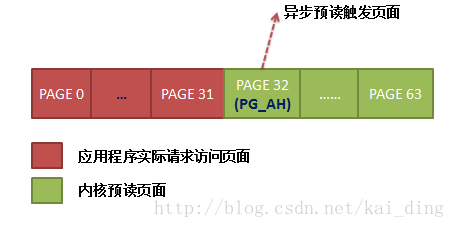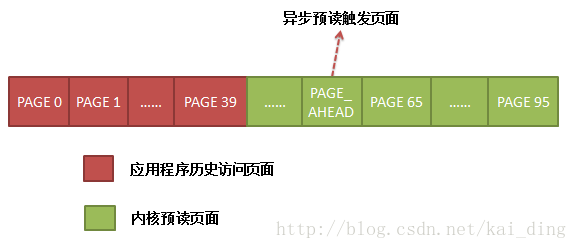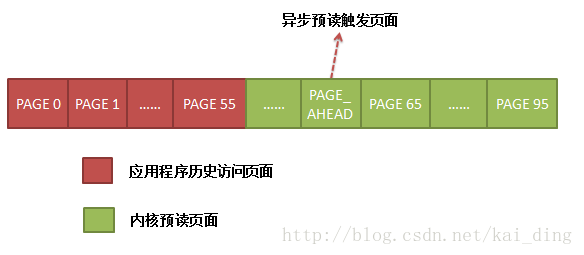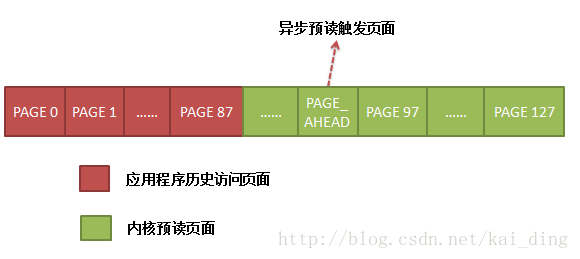# Linux文件系统预读(二)

#### 情境2

``````{
...
f   = open("file", ....);
ret = read(f, buf, 40 * 4096);
ret = read(f, buf, 16 * 4096);
ret = read(f, buf, 32 * 4096);
...
}
``````

``````initial_readahead:
ra->start = offset;
ra->size = get_init_ra_size(req_size, max);
// ra->size 一定是>= req_size的，这个由get_init_ra_size保证
// 如果req_size >= max,那么ra->async_size = ra_size
ra->async_size = ra->size > req_size ? ra->size - req_size : ra->size;

/*
*/
if (offset == ra->start && ra->size == ra->async_size) {
ra->async_size = get_next_ra_size(ra, max);
ra->size += ra->async_size;
}

return ra_submit(ra, mapping, filp);
}
````````````     /* 如果:
** 1. 顺序读(本次读偏移为上次读偏移 (ra->start) + 读大小(ra->size,包含预读量) -
**    上次预读大小(ra->async_size))
** 2. offset == (ra->start + ra->size)???
*/
if ((offset == (ra->start + ra->size - ra->async_size) ||
offset == (ra->start + ra->size))) {
// 设置本次读的
ra->start += ra->size;
ra->size = get_next_ra_size(ra, max);
ra->async_size = ra->size;
}
``````